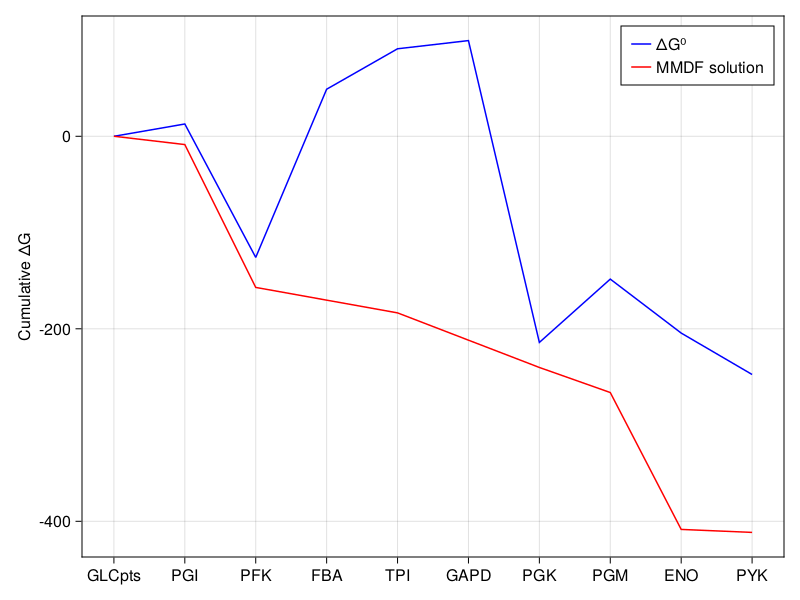# Maximum-minimum driving force analysis

Here, we use the max-min driving force analysis (MMDF) to find optimal concentrations for the metabolites in glycolysis to ensure that the smallest driving force across all the reactions in the model is as large as possible. The method is described in more detail by Flamholz, et al., "Glycolytic strategy as a tradeoff between energy yield and protein cost.", Proceedings of the National Academy of Sciences, 2013.

using COBREXA, GLPK

!isfile("e_coli_core.json") &&

model = load_model("e_coli_core.json")
Metabolic model of type JSONModel
sparse([9, 51, 55, 64, 65, 34, 44, 59, 66, 64  …  20, 22, 23, 25, 16, 17, 34, 44, 57, 59], [1, 1, 1, 1, 1, 2, 2, 2, 2, 3  …  93, 93, 94, 94, 95, 95, 95, 95, 95, 95], [1.0, 1.0, -1.0, -1.0, 1.0, -1.0, 1.0, -1.0, 1.0, 1.0  …  1.0, -1.0, 1.0, -1.0, -1.0, 1.0, -1.0, 1.0, 1.0, -1.0], 72, 95)
Number of reactions: 95
Number of metabolites: 72


For MMDF to work, we need thermodynamic data about the involved reactions.

In particular, we will use reaction Gibbs free energies (ΔG⁰) that can be obtained e.g. from eQuilibrator (possibly using the existing Julia wrapper that allows you to automate this step in Julia).

Here, we have gathered a dictionary that maps the reaction IDs to calculated Gibbs free energy of reaction for each metabolic reaction (including the transporters). The units of the measurements are not crucial for the computation, but we use the usual kJ/mol for consistency.

reaction_standard_gibbs_free_energies = Dict(
"ACALD" => -21.26,
"PTAr" => 8.65,
"ALCD2x" => 17.47,
"PDH" => -34.24,
"PYK" => -24.48,
"CO2t" => 0.00,
"MALt2_2" => -6.83,
"CS" => -39.33,
"PGM" => -4.47,
"TKT1" => -1.49,
"ACONTa" => 8.46,
"GLNS" => -15.77,
"ICL" => 9.53,
"FBA" => 23.37,
"SUCCt3" => -43.97,
"FORt2" => -3.42,
"G6PDH2r" => -7.39,
"AKGDH" => -28.23,
"TKT2" => -10.31,
"FRD7" => 73.61,
"SUCOAS" => -1.15,
"FBP" => -11.60,
"ICDHyr" => 5.39,
"AKGt2r" => 10.08,
"GLUSy" => -47.21,
"TPI" => 5.62,
"FORt" => 13.50,
"ACONTb" => -1.62,
"GLNabc" => -30.19,
"RPE" => -3.38,
"ACKr" => 14.02,
"THD2" => -33.84,
"PFL" => -19.81,
"RPI" => 4.47,
"D_LACt2" => -3.42,
"TALA" => -0.94,
"PPCK" => 10.65,
"ACt2r" => -3.41,
"NH4t" => -13.60,
"PGL" => -25.94,
"PGK" => 19.57,
"LDH_D" => 20.04,
"ME1" => 12.08,
"PIt2r" => 10.41,
"ATPS4r" => -37.57,
"PYRt2" => -3.42,
"GLCpts" => -45.42,
"GLUDy" => 32.83,
"CYTBD" => -59.70,
"FUMt2_2" => -6.84,
"FRUpts2" => -42.67,
"GAPD" => 0.53,
"H2Ot" => 0.00,
"PPC" => -40.81,
"PFK" => -18.54,
"MDH" => 25.91,
"PGI" => 2.63,
"O2t" => 0.00,
"ME2" => 12.09,
"GND" => 10.31,
"SUCCt2_2" => -6.82,
"GLUN" => -14.38,
"ETOHt2r" => -16.93,
"ACALDt" => 0.00,
"SUCDi" => -73.61,
"ENO" => -3.81,
"MALS" => -39.22,
"GLUt2r" => -3.49,
"PPS" => -6.05,
"FUM" => -3.42,
);

COBREXA implementation of MMDF enforces that ΔᵣG .* v ≤ 0 (where v is the flux solution). This is slightly less restrictive than the original formulation of MMDF, where all fluxes are enforced to be positive; instead, the COBREXA solution needs a pre-existing thermodynamically consistent solution that is used as a reference.

We can generate a well-suited reference solution using e.g. the loopless FBA:

flux_solution = flux_balance_analysis_dict(
model,
GLPK.Optimizer;
)
Dict{String, Float64} with 95 entries:
"ACALD"       => 0.0
"PTAr"        => 0.0
"ALCD2x"      => 0.0
"PDH"         => 9.28253
"PYK"         => 1.75818
"CO2t"        => -22.8098
"EX_nh4_e"    => -4.76532
"MALt2_2"     => 0.0
"CS"          => 6.00725
"PGM"         => -14.7161
"TKT1"        => 1.49698
"EX_mal__L_e" => 0.0
"ACONTa"      => 6.00725
"EX_pi_e"     => -3.2149
"GLNS"        => 0.223462
"ICL"         => 0.0
"EX_o2_e"     => -21.7995
"FBA"         => 7.47738
"EX_gln__L_e" => 0.0
⋮             => ⋮

We can now run the MMDF.

In the call, we specify the metabolite IDs of protons and water so that they are omitted from concentration calculations. Also, the water transport reaction should typically also be ignored. Additionally, we can fix the concentration ratios of certain metabolites directly.

The reason for removing the protons and water from the concentration calculations is because the Gibbs free energies of biochemical reactions are measured at constant pH in aqueous environments. Allowing the model to change the pH would break the assumptions about validity of the thermodynamic measurements.

sol = max_min_driving_force(
model,
reaction_standard_gibbs_free_energies,
GLPK.Optimizer;
flux_solution = flux_solution,
proton_ids = ["h_c", "h_e"],
water_ids = ["h2o_c", "h2o_e"],
concentration_ratios = Dict(
),
concentration_lb = 1e-6, # 1 uM
concentration_ub = 0.1, # 100 mM
ignore_reaction_ids = [
"H2Ot", # ignore water transport
],
)

sol.mmdf
1.7695541754085948
Note: transporters

Transporters can be included in MMDF analysis, however water and proton transporters must be excluded explicitly in ignore_reaction_ids. In turn, the ΔᵣG for these transport reactions will always be 0. If you do not exclude the transport of the metabolites, the MMDF will likely only have a zero solution.

Finally, we show how the concentrations are optimized to ensure that each reaction proceeds "down the hill" (ΔᵣG < 0). We can explore the glycolysis pathway reactions:

glycolysis_pathway =
["GLCpts", "PGI", "PFK", "FBA", "TPI", "GAPD", "PGK", "PGM", "ENO", "PYK"]
10-element Vector{String}:
"GLCpts"
"PGI"
"PFK"
"FBA"
"TPI"
"GAPD"
"PGK"
"PGM"
"ENO"
"PYK"

We additionally scale the fluxes according to their stoichiometry in the pathway. From the output, we can clearly see that metabolite concentrations play a large role in ensuring the thermodynamic consistency of in vivo reactions.

using CairoMakie

standard_dg = cumsum([
reaction_standard_gibbs_free_energies[rid] * flux_solution[rid] for
rid in glycolysis_pathway
]);
optimal_dg =
cumsum([sol.dg_reactions[rid] * flux_solution[rid] for rid in glycolysis_pathway]);

f = Figure();
ax = Axis(f[1, 1], ylabel = "Cumulative ΔG", xticks = (1:10, glycolysis_pathway));
lines!(ax, 1:10, standard_dg .- first(standard_dg), color = :blue, label = "ΔG⁰");
lines!(ax, 1:10, optimal_dg .- first(optimal_dg), color = :red, label = "MMDF solution");
axislegend(ax)
fThermodynamic variability

As with normal flux variability, thermodynamic constraints in a model also allow a certain amount of parameter selection freedom. Specialized max_min_driving_force_variability can be used to explore the thermodynamic solution space more easily.